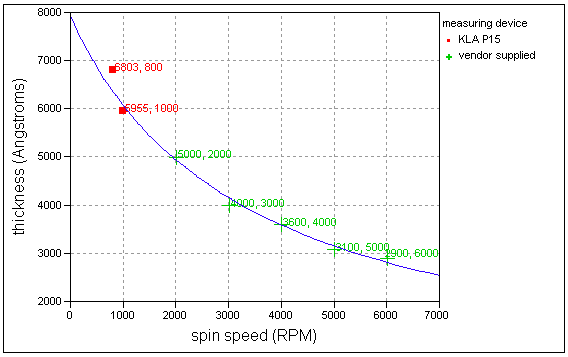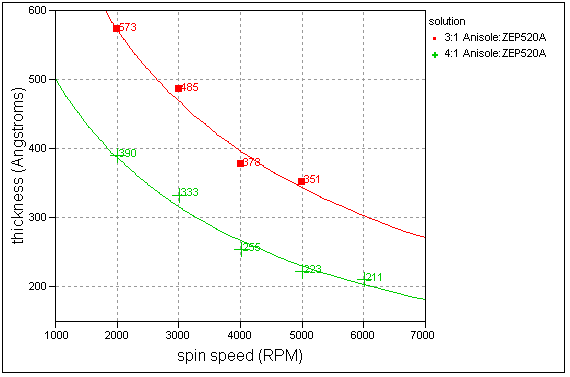HOME   •  SERVICES   •  PROCESSES   •  LINKS   •  CONTACTS

ZEP520A thickness vs. spin speed curves
(1/y) = 1.259E-3 + (3.8E-8)xAnisole:ZEP520A 1:1 dilution thickness vs. spin speed
3000 RPM, 1500RPM/s, 60sec on 4" wafer
mean = 1264 A, sigma = 11 A, N = 6

Anisole:ZEP520A 2:1 dilution thickness vs. spin speed curve
(1/y) = 5.48E-4 + (1.38E-7)xAnisole:ZEP520A 3:1, 4:1 dilution thickness vs. spin speed curves
3:1 solution curve fit: (1/y) = 9.63E-4 + (3.88E-7)x
4:1 solution curve fit: (1/y) = 1.41E-3 + (5.82E-7)x